Name:    MCAS Distance-Displacement, Speed-Velocity 2004-2014

Multiple Choice
Identify the choice that best completes the statement or answers the question.

1.

An elevator in an office building completed the following trips:

• 1st floor to 8th floor
• 8th floor to 4th floor
• 4th floor to 13th floor

The distance between each floorof the office building is 3.0 m.
Which table shows the total distance traveled and displacement of the elevator? (MCAS 2010)
 a.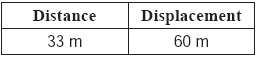b.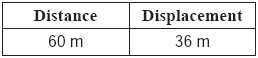c.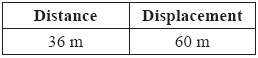d.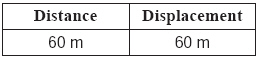2.

A racecar travels at an average speed of 80 m/s in a race. The total distance of the race is 640,000 m. How long does it take the racecar to finish the race? (MCAS 2011)
 a. 800 s c. 6,400,000 s b. 8,000 s d. 51,200,000 s

3.

Two soccer players, X and Y, are kicking a ball back and forth to each other, as shown below. (MCAS 2012)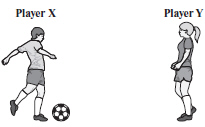The table below shows the distance and direction the ball moves after each of four kicks.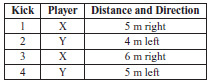What is the total displacement of the ball and the total distance traveled by the ball?
 a. The ball has a displacement of 2 m to the right and traveled a distance of 20 m. b. The ball has a displacement of 20 m to the right and traveled a distance of 2 m. c. The ball has a displacement of 9 m to the right and traveled a distance of 11 m. d. The ball has a displacement of 11 m to the right and traveled a distance of 9 m.

4.

A person starts driving and travels 3 km east to a store. The person then turns around and travels 1 km west to another store. Finally, the person travels 2 km west, back to the starting point. What distance has this person traveled? (MCAS 2013)
 a. 0 km c. 5 km b. 3 km d. 6 km

5.

A person runs 3 km north. One hour later, she runs 3 km south. Which of the following statements describes the person’s complete trip? (MCAS 2014)
 a. The displacement is 3 km. b. The distance traveled is 3 km. c. The distance traveled is greater than the displacement. d. The displacement is greater than the distance traveled.

Essay

6.

A person is driving his car to a store. The store is 800 m north of the person’s starting point. After traveling north 1200 m, the person realizes that he has passed the store. He turns the car around and drives back to the store. Altogether, the trip to the store takes 100 s. (MCAS 2011)

a.       Determine the distance traveled by the car on this trip to the store. Show your calculations and include units in your answer.

b.       Calculate the average speed of the car on this trip. Show your calculations and include units in your answer.

c.       Determine the displacement of the car for this trip. Include units in your answer.

d.       Calculate the average velocity of the car upon arrival at the store. Show your calculations and include units in your answer.

7.

A student leaves school and walks south, arriving home after 18 min. The distance between the school and her home is 1200 m. At home, the student realizes she left a book at school. She walks back, arriving at the school 18 min later. (MCAS 2013)
a. Determine the student’s average speed as she walks home. Show your calculations and include units in your answer.
b. Describe the difference between the student’s average velocity walking home and her average velocity walking back to the school.
c. Calculate the total distance the student walked. Show your calculations and include units in your answer.
d. Determine the final displacement of the student. Explain your answer.